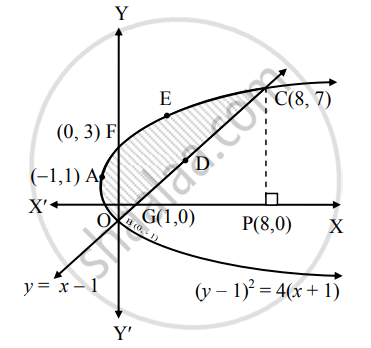# Find the area of the region bounded by the curve (y − 1)2 = 4(x + 1) and the line y = (x − 1) - Mathematics and Statistics

Sum

Find the area of the region bounded by the curve (y − 1)2 = 4(x + 1) and the line y = (x − 1)

#### Solution

Given equation of the curve is

(y − 1)2 = 4(x + 1)     .......(i)

This is a parabola with vertex at A(−1, 1).

and equation of the line is y = x − 1   ......(ii)

Find the points of intersection of (y − 1)2 = 4(x + 1) and y = (x − 1)

Substituting (ii) in (i), we get

(x − 1 − 1)2 = 4(x + 1)

∴ x2 − 4x + 4 = 4x + 4

∴ x2 − 8x = 0

∴ x(x − 8) = 0

∴ x = 0 or x = 8

When x = 0, y = 0 − 1 = −1 and

when x = 8, y = 8 − 1 = 7

∴ The points of intersection are B(0, −1) and C(8, 7).

To find the points where the parabola

(y − 1)2 = 4(x + 1) cuts the Y-axis,

Substituting x = 0 in (i), we get

(y − 1)2 = 4(0 + 1) = 4

∴ y − 1 = ± 2

∴ y − 1 = 2 or y − 1 = −2

∴ y = 3 or y = −1

∴ The parabola cuts the Y-axis at the points B(0, −1) and F(0, 3).

To find the point where the line y = x − 1 cuts the X-axis, Substituting y = 0 in (ii), we get

x − 1 = 0

∴ x = 1

∴ The line cuts the X-axis at the point G(1, 0).Required area = area of the region BFAB + area of the region OGDCEFO + area of the region OBGO

Now, area of the region BFAB

= area under the parabola (y − 1)2 = 4(x + 1),

Y-axis from y = −1 to y = 3

= int_(-1)^3 x  "d"y

= int_(-1)^3[(y - 1)^2/4 - 1] "d"y    ......[Form (i)]

= [1/4*(y - 1)^3/3 - y]_(-1)^3

= [1/12 (3 - 1)^3 - 3] - [1/12(-1 - 1)^3 − (-1)]

= 8/13 - 3 + 8/12 - 1

= 4/3 - 4

= -8/3

= 8/3    .......[∵ area cannot be negative]

Area of the region OGDCEFO = area of the region OPCEFO − area of the region GPCDG

= int_0^8 y  "d"x - int_1^8 y  "d"x

= int_0^8 (2sqrt(x + 1) + 1) "d"x - int_1^8(x -1)  "d"x   ......[From (i) and (ii)]

= [2*((x + 1)^(3/2))/(3/2) + x]_0^8 - [x^2/2 - x]_1^8

= [4/(9)^(3/2) + 8 - 4/3(1)^(3/2) - 0] - [(64/2 - 8) - (1/2 - 1)]

= (36 + 8 - 4/3) - (24 + 1/2)

= 44 - 4/3 - 24 - 1/2

= 20 - (4/3 + 1/2)

= 20 - 11/6

= 109/6

Area of the region OBGO = int_0^1 y  "d"x

= int_0^1(x - 1)  "d"x    ......[From (ii)]

= [x^2/2 - x]_0^1

= 1/2 - 1 - 0

= 1/2    ......[∵ area cannot be negative]

∴ Required area = 8/3 + 109/6 + 1/2

= (16 + 109 + 3)/6

= 64/3 sq.units

Concept: Area Bounded by the Curve, Axis and Line
Is there an error in this question or solution?